### IMO Shortlist 2018 problem N2

Kvaliteta:
Avg: 0,0
Težina:
Avg: 6,0

Let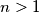$n>1$ be a positive integer. Each cell of an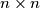$n\times n$ table contains an integer. Suppose that the following conditions are satisfied:

(i) Each number in the table is congruent to$1$ modulo$n$;

(ii) The sum of numbers in any row, as well as the sum of numbers in any column, is congruent to$n$ modulo$n^2$.

Let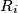$R_i$ be the product of the numbers in the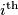$i^{\text{th}}$ row, and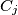$C_j$ be the product of the number in the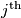$j^{\text{th}}$ column. Prove that the sums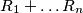$R_1+\hdots R_n$ and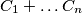$C_1+\hdots C_n$ are congruent modulo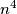$n^4$.

Izvor: https://www.imo-official.org/problems/IMO2018SL.pdf